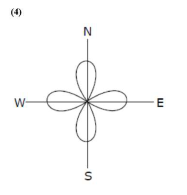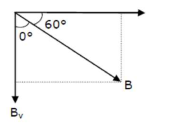# An aeroplane, with its wings spread 10 m,

Question:

An aeroplane, with its wings spread $10 \mathrm{~m}$, is flying at a speed of $180 \mathrm{~km} / \mathrm{h}$ in a horizontal direction. The total intensity of earth's field at that part is $2.5 \times 10^{-4} \mathrm{~Wb} / \mathrm{m}^{2}$ and the angle of dip is $60^{\circ}$. The emf induced between the tips of the plane wings will be

1. (1) $88.37 \mathrm{mV}$

2. (2) $62.50 \mathrm{mV}$

3. (3) $54.125 \mathrm{mV}$

4. (4) $108.25 \mathrm{mV}$

Correct Option: , 4

Solution:$\sum=B \perp v \xi$

$\sin 60^{\circ}-\frac{B_{n}}{u}$$\frac{\sqrt{3}}{2}=\frac{B_{3}}{B}$

$B V=\frac{\sqrt{3}}{2} B$

$E=\frac{\sqrt{3}}{2} B \ell v$

$=\frac{\sqrt{3}}{2} \times 2.5 \times 10^{-4} \times 10 \times 180 \times \frac{5}{18}$

$=\frac{\sqrt{3}}{2} \times 2.5 \times 5 \times 10^{-2}=10.825 \times 10^{-2}=108.25 \mathrm{mV}$